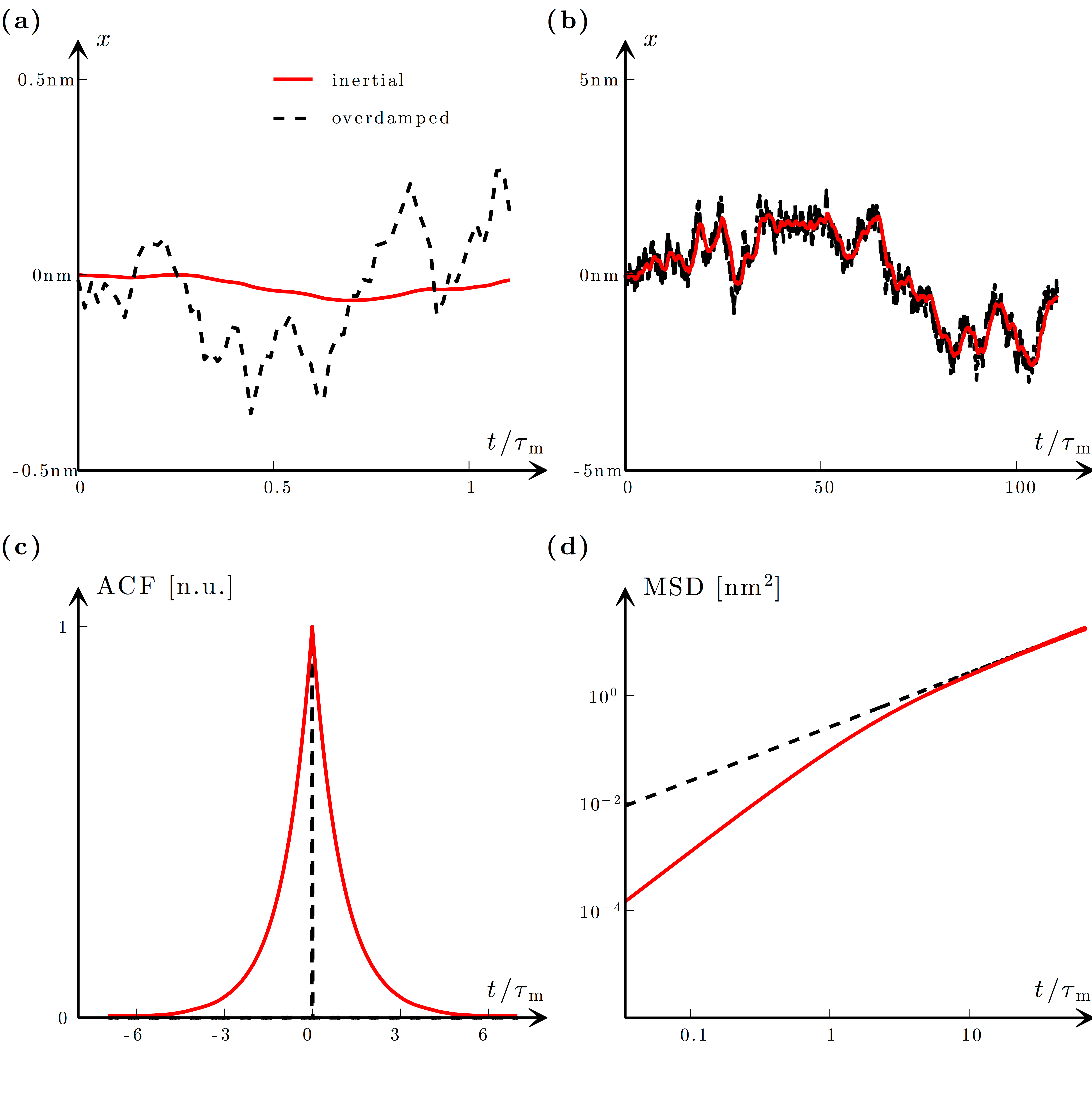# Figure 7.8 — Inertial and diffusive regimesFigure 7.8 — Inertial and diffusive regimes. (a) For times smaller than or comparable to the inertial time τm the trajectory of a particle with inertia (solid line) appears smooth. In contrast, in the absence of inertia (dashed line) the trajectory is ragged and discontinuous. (b) For times significantly longer than τm, both the trajectory with inertia (solid line) and that without inertia (dashed line) are jagged, because the microscopic details are not resolvable. These trajectories are computed using Eqs. (7.29) and (7.30) with Δt = 10 ns and the same realisation of white noise so that the two trajectories can be compared. (c) The velocity autocorrelation function (ACF) for a particle with inertia (solid line) decays to zero with the time constant τm, whereas that for a particle without inertia (dashed line) drops immediately to zero, demonstrating that the particle velocity is not correlated and does not have a characteristic time scale. (d) A log–log plot of the mean squared displacement (MSD) for a particle with inertia (solid line) shows a transition from quadratic behaviour at short times to linear behaviour at long times, whereas for a particle without inertia (dashed line) it is always linear. The simulation parameters are the same as in Fig. 7.6.Adapted with permission from Volpe and Volpe, Am. J. Phys. 81, 224–30. Copyright 2013, American Association of Physics Teachers.